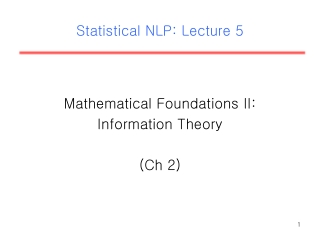DownloadDownload PresentationStatistical NLP: Lecture 5

# Statistical NLP: Lecture 5

Télécharger la présentation## Statistical NLP: Lecture 5

- - - - - - - - - - - - - - - - - - - - - - - - - - - E N D - - - - - - - - - - - - - - - - - - - - - - - - - - -
##### Presentation Transcript

1. Statistical NLP: Lecture 5 Mathematical Foundations II: Information Theory (Ch 2)

2. Entropy • The entropy is the average uncertainty of a single random variable. • Let p(x)=P(X=x); where x ∈X. • H(p)= H(X)= - Σx∈Xp(x)log2p(x) • In other words, entropy measures the amount of information in a random variable. It is normally measured in bits.

3. Joint Entropy and Conditional Entropy • The joint entropyof a pair of discrete random variables X, Y ~ p(x,y) is the amount of information needed on average to specify both their values. • H(X,Y) = - Σx∈XΣy∈Y p(x,y)log2 p(x,y) • The conditional entropyof a discrete random variable Y given another X, for X, Y ~ p(x,y), expresses how much extra information you still need to supply on average to communicate Y given that the other party knows X. • H(Y|X) = - Σx∈XΣy∈Y p(x,y)log2p(y|x) • Chain Rulefor Entropy: H(X,Y)=H(X)+H(Y|X)

4. Mutual Information • By the chain rule for entropy, we have H(X,Y) = H(X)+ H(Y|X) = H(Y)+H(X|Y) • Therefore, H(X)-H(X|Y)=H(Y)-H(Y|X) • This difference is called the mutual information between X and Y. • It is the reduction in uncertainty of one random variable due to knowing about another, or, in other words, the amount of information one random variable contains about another.

5. The Noisy Channel Model • Assuming that you want to communicate messages over a channel of restricted capacity, optimize (in terms of throughput and accuracy) the communication in the presence of noise in the channel. • A channel’s capacity can be reached by designing an input code that maximizes the mutual information between the input and output over all possible input distributions. • This model can be applied to NLP.

6. Relative Entropy or Kullback-LeiblerDivergence • For 2 pmfs, p(x) and q(x), their relative entropyis: • D(p||q)= Σx∈X p(x)log(p(x)/q(x)) • The relative entropy (also known as the Kullback- Leibler divergence) is a measure of how different two probability distributions (over the same event space) are. • The KL divergence between p and q can also be seen as the average number of bits that are wasted by encoding events from a distribution p with a code based on a not-quite-right distribution q.

7. The Relation to Language:Cross-Entropy • Entropy can be thought of as a matter of how surprised we will be to see the next word given previous words we already saw. • The cross entropybetween a random variable X with true probability distribution p(x) and another pmf q (normally a model of p) is given by: H(X,q)=H(X)+D(p||q). • Cross-entropy can help us find out what our average surprise for the next word is.

8. The Entropy of English • We can model English using n-gram models(also known a Markov chains). • These models assume limited memory, i.e., we assume that the next word depends only on the previous k ones [kth order Markov approximation]. • What is the Entropy of English?

9. Perplexity • A measure related to the notion of cross-entropy and used in the speech recognition community is called the perplexity. • Perplexity(x1n, m) =2H(x1n,m) =m(x1n)-1/n • A perplexity of k means that you are as surprised on average as you would have been if you had had to guess between k equiprobable choices at each step.